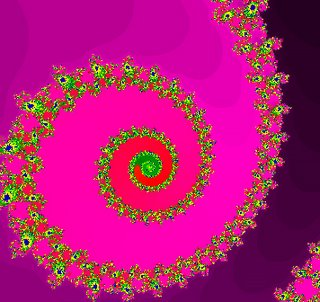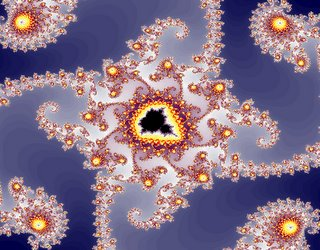## Wednesday, March 08, 2006

### What the fuck is a Mandelbrot set?I had a vague idea that these existed because of the picture of a nautilus on the cover of my calculus textbook. It's the shapes that are defined by plugging different values or colors into the "recurrence" equationThere was a show about PBS last night about it, called "Colours of Infinity." This is one of those instances where, you instinctively know something on a metaphysical or psychic level for, say, a few thousand years, and then some scientists or mathematicians come along and are able to explain it in terms that make sense to them. Because some of us are not linear but abstract thinker-feelers, we don't necessarily need these tools to feel moved and understand a meaning behind the beautiful chaos that is life. But the linear-thinking types do need them, and so we get these groovy pictures to set as wallpaper on our monitors.

I'm sure I can't explain it as well as they did on the show, so, thanks to the nearly limitless font of information that is the wild wacky web, I'll copy what I think is the gist of the thing from Wikipedia:

## Periodic cycles in the Mandelbrot set

Inside the Mandelbrot set, the iteration of sequence zn+1= zn2+c evolve in different ways for different values of c. For values of c inside the big cardioid, the iterations converge to a point. For c inside the big bulb to the left of the cardioid, the iterations converge to a cycle of period 2. For other bulbs, the iterations converge to a cycle of a different period n, according to the numbers shown in the following figure.

Notice that in the bulb in the middle of bulb with n=2 and the bulb with n=3, the period of the cycle is n=2+3=5;in the bulb in the middle of the bulb with n=2 and the bulb with n=5, the period of the cycle is n=2+5=7; in the bulb in the middle of the bulb with n=2 and the bulb with n=7, the period of the cycle is n=2+7=9. In fact, there are so many bulbs as the real numbers between 2 and 3 in that quadrant of the main bulb!

The number of rays coming out of the antena of each bulb corresponds to the period of the cycle that bulb. In the corresponding Julia sets, the number of rays also corresponds to the period of the cycle the corresponding bulb.

If we look at the trajectory of the periodic cycle in a bulb with n=5, we see that the cycle jumps always 2 components counterclokwise, in each iteration (i.e. the cycle rotates by 2/5 of a revolution, in each iteration). And the same happens in the corresponding Julia set.

So the bulbs with n=2,7,5,8,3,7,4,5,6,7 in the figure can be represented instead by the rotation parameters r=1/2, 3/7, 2/5, 3/8, 1/3, 2/7, 1/4, 1/5, 1/6, 1/7, which are ordered as a sequence of decreasing rational numbers.

Notice that the bulb in the middle of the bulb with r=2/5 and the bulb with r=1/2, r=2/5+1/2=3/7; and in the middle of the bulbs with r==3/7 and 1/2, there is also a bulb with r=4/9, etc... There is a bulb with r=p/q for each rational number between 0 and 1, and they are correctly ordered around the cardioid in a counterclockwise fashion. In the bottom part of the Mandelbrot set, the cycles rotate clockwise.

### Adding color

Mathematically speaking, the pictures of the Mandelbrot set and Julia sets are "black and white". Either a point is in the set or it is not. Most computer-generated graphs are drawn in color. Under the most common rendering method, for the points that diverge to infinity, and are therefore not in the set, the color reflects the number of iterations it takes to reach a certain distance from the origin. This creates concentric shapes, each a better approximation to the Mandelbrot set than the last. One possible scheme is that points that diverge quickly are drawn in black; then you have brighter colors for the middle; then you have white for the points in the set, and near-white for the points that diverge very slowly.

***********************************************************************************

Got that?

#### 1 comment:

Amaha said...

I am absolutely clueless! I will say, however, I love the term "Colours of Infinity."